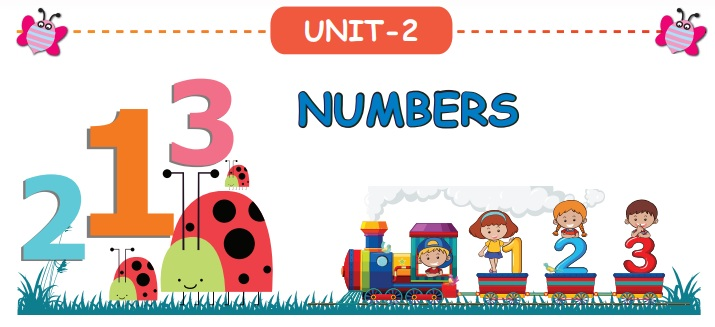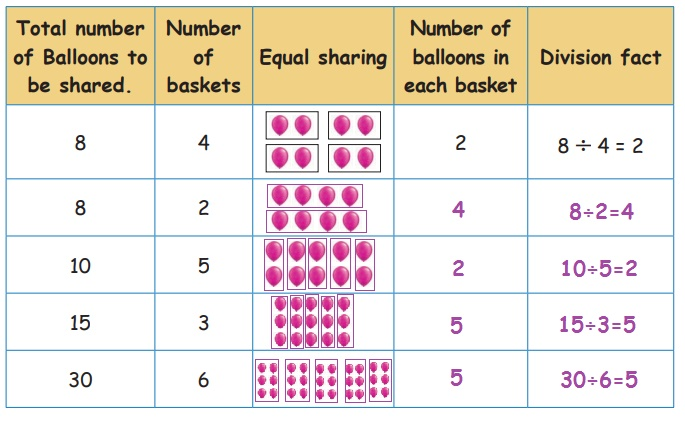Home | | Maths 3rd Std | Equal sharing and repeated subtraction

# Equal sharing and repeated subtraction

Equal sharing is mathematically called as "division" and division is denoted by the symbol "÷".

UNIT−2

NUMBERSEqual sharing and repeated subtraction

Kabilan had 30 mangoes and wanted to share them among 5 of his friends. Let us see the way he shared the one by one mangoes equally among his friends.F = Friend

Number of steps = 6

Let us express this in a number lineRepeated subtraction statement 30 − 5 − 5 −5 − 5 −5 −5 = 0

Kabilan distributed 30 mangoes among 5 of his friends by giving them one mango in each step. Thus each friend has got 6 mangoes.

Imagine Kabilan has to share these 30 mangoes among 10 of his friends.Number of steps = 3

The number line for the above situation:Repeated subtraction statement 30 −10 −10 − 10 = 0

This time Kabilan has shared 30 mangoes among 10 of his friends in 3 steps. Each friends has got 3 mangoes.

Suppose Kabilan has to share the mangoes among 15 of his friends. How many mangoes would each of them get? How Many steps would he require to share the mangoes among them?Number of steps = 2

Complete the number lineRepeated subtraction statement 30 – 15 – 15 = 0

Kabilan shared 30 mangoes among 15 of his friends in 2 steps.

Each friend got 2 mangoes.

Let us summarize the above 3 examples as follows.This can also be written as

30 ÷ 5 = 6

30 ÷ 10 = 3

30 ÷ 15 = 2Equal sharing is mathematically called as "division" and division is denoted by the symbol "÷".Let us see another example

8 ÷ 4 = 2

Here, 8 is the dividend 4 is the divisor and 2 is the quotient

Complete the table.Can you share 2 mangoes among 5 of your friends?

No, This means that dividend should always be greater than the divisor.

Tags : Numbers | Term 3 Chapter 2 | 3rd Maths , 3rd Maths : Term 3 Unit 2 : Numbers
Study Material, Lecturing Notes, Assignment, Reference, Wiki description explanation, brief detail
3rd Maths : Term 3 Unit 2 : Numbers : Equal sharing and repeated subtraction | Numbers | Term 3 Chapter 2 | 3rd Maths